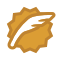# LeetCode 15三数之和&16最接近的三数之和

## 三数之和(双指针)

[
[-1, 0, 1],
[-1, -1, 2]
]

public List<List<Integer>> threeSum(int[] nums) {
List<List<Integer>> list=new ArrayList<List<Integer>>();
Set<String>set=new HashSet<String>();
Arrays.sort(nums);

for(int i=1;i<nums.length-1;i++)
{
int left=0;int right=nums.length-1;

while (left<i&&right>i) {
//System.out.println(i+" "+left+" "+i+" "+right+" "+list.toString());
if(nums[left]>0)break;
int sum=nums[left]+nums[i]+nums[right];
//System.out.println(nums[left]+" "+nums[i]+" "+nums[right]);
if(sum==0)
{

String teamString=nums[left]+" "+nums[i]+" "+nums[right];
if(!set.contains(teamString)) {
List<Integer>list2=new ArrayList<Integer>();
}
left++;right--;
}
else if (sum>0) {
right--;
}
else {
left++;
}
}

}
return list;
}


• 当前最左侧数字sums[i]如果和sums[i-1]（i>1）相等，那么跳出本次计算，因为当前数字如果作为最左侧数字那么前面有相同的数字可以组成这个集合，所以不需要这部的计算
• 同时在确定最左侧，中间left和最右侧right向中间进行时如果三数之和大于0，那么right–；如果三数之和小于0，那么left++；同时你可以进行部分剪枝优化，把不可能的情况直接过滤掉。

ac代码为：

 public List<List<Integer>> threeSum(int[] nums) {
List<List<Integer>> list=new ArrayList<List<Integer>>();
Arrays.sort(nums);
for(int i=0;i<nums.length-2;i++)
{
int left=i+1;int right=nums.length-1;
if(i>0&&nums[i]==nums[i-1])continue;
while (left<right) {
//System.out.println(i+" "+left+" "+i+" "+right+" "+list.toString());
if(nums[left]+nums[i]>0)break;
int sum=nums[left]+nums[i]+nums[right];
//System.out.println(nums[left]+" "+nums[i]+" "+nums[right]);
if(sum==0)
{
List<Integer>list2=new ArrayList<Integer>();
while (left<right&&nums[left]==nums[left+1]) {left++;}
while (left<right&&nums[right]==nums[right-1]) {right--;}
left++;right--;
}
else if (sum>0) {
right--;
}
else {
left++;
}
}

}
return list;
}


## 最接近的三数之和

3 <= nums.length <= 10^3
-10^3 <= nums[i] <= 10^3
-10^4 <= target <= 10^4

 public static int threeSumClosest(int[] nums, int target) {
Arrays.sort(nums);
int value=nums+nums+nums;
for(int i=0;i<nums.length-2;i++)
{
int left=i+1;int right=nums.length-1;

while (left<right) {
int sum=nums[left]+nums[i]+nums[right];
int gap1=Math.abs(value-target);
int gap2=Math.abs(sum-target);
if(target>=0&&nums[left]+nums[i]-target>gap1)break;
if(gap2<gap1)
{
value=sum;
}
if (sum==target) {
return target;
}
else if(sum>target) {
right--;
}
else {
left++;
}
}
}
return value;
}Big saiCSDN认证博客专家 scikit-learn

03-1864410-201961
04-05833
09-26758
03-19689
06-072万+
09-16507
07-065554
09-18605
09-17601